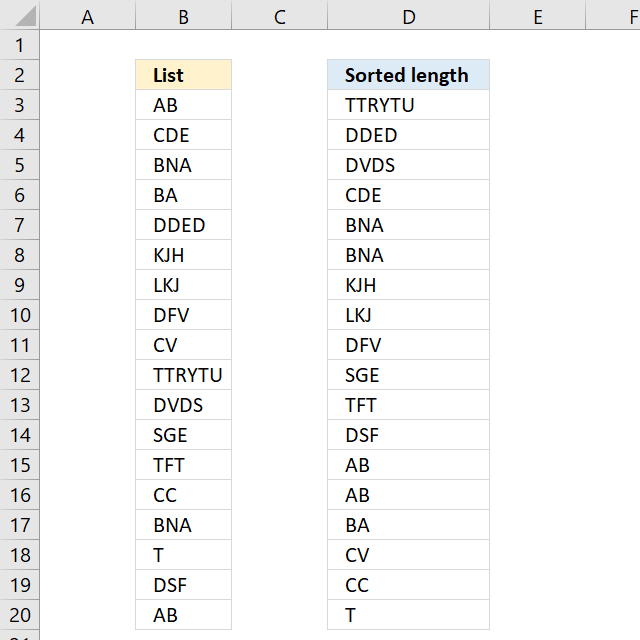Author: Oscar Cronquist Article last updated on October 02, 2022The image above demonstrates a formula in cell D3 that sorts values based on character length, the value with the most characters are at the top, and the value with the least amount of characters is at the bottom.

I recommend Excel 365 users this article: Sort by word length. That formula is considerably smaller and easier to understand.

Array formula in D3:

=INDEX(\$B\$3:\$B\$20, MATCH(LARGE(LEN(\$B\$3:\$B\$20), ROWS(\$A\$1:A1)), LEN(\$B\$3:\$B\$20)*(COUNTIF(\$F\$2:F2, \$B\$3:\$B\$20)<COUNTIF(\$B\$3:\$B\$20, \$B\$3:\$B\$20)), 0))

copied down as far as needed.

To enter an array formula, type the formula in a cell then press and hold CTRL + SHIFT simultaneously, now press Enter once. Release all keys.

The formula bar now shows the formula with a beginning and ending curly bracket telling you that you entered the formula successfully. Don't enter the curly brackets yourself.

### Explaining formula in cell B2

#### Step 1 - Calculate the k-th largest string length

In order to return a new value in each cell, the formula uses an expanding cell reference to return the next largest value. The LEN function returns the character length of a value, the ROWS function returns the number of rows in a cell reference. The LARGE function returns the k-th largest value. LARGE( array, k)

LARGE(LEN(\$B\$3:\$B\$20), ROWS(\$A\$1:A1))

becomes

LARGE(LEN(\$B\$3:\$B\$20), 1)

becomes

LARGE({2; 3; 3; 2; 4; 3; 3; 3; 2; 6; 4; 3; 3; 2; 3; 1; 3; 2}, 1)

and returns 6.

#### Step 2 - Check previously displayed values in cells above

The COUNTIF function counts cells in cell range based on a condition or criteria. The first argument contains an expanding cell reference that lets the formula keep track of already shown values.

COUNTIF(\$F\$2:F2, \$B\$3:\$B\$20)

becomes

COUNTIF("Sorted length",{"AB"; "CDE"; "BNA"; "BA"; "DDED"; "KJH"; "LKJ"; "DFV"; "CV"; "TTRYTU"; "DVDS"; "SGE"; "TFT"; "CC"; "BNA"; "T"; "DSF"; "AB"})

and returns

{0;0;0;0;0;0;0;0;0;0;0;0;0;0;0;0;0;0}

#### Step 3 - Check if the count of prior values are less than the total count of each value

(COUNTIF(\$D\$2:D2,\$B\$3:\$B\$20)<COUNTIF(\$B\$3:\$B\$20,\$B\$3:\$B\$20)

becomes

{0; 0; 0; 0; 0; 0; 0; 0; 0; 0; 0; 0; 0; 0; 0; 0; 0; 0}<{2; 1; 2; 1; 1; 1; 1; 1; 1; 1; 1; 1; 1; 1; 2; 1; 1; 2}

and returns

{TRUE; TRUE; TRUE; TRUE; TRUE; TRUE; TRUE; TRUE; TRUE; TRUE; TRUE; TRUE; TRUE; TRUE; TRUE; TRUE; TRUE; TRUE}.

#### Step 4 - Match length to array

The MATCH function returns the relative position in a cell range or array of a given value.

MATCH(LARGE(LEN(\$B\$3:\$B\$20), ROWS(\$A\$1:A1)), LEN(\$B\$3:\$B\$20)*(COUNTIF(\$F\$2:F2, \$B\$3:\$B\$20)<COUNTIF(\$B\$3:\$B\$20, \$B\$3:\$B\$20)), 0)

becomes

MATCH(6, LEN(\$B\$3:\$B\$20)*(COUNTIF(\$F\$2:F2, \$B\$3:\$B\$20)<COUNTIF(\$B\$3:\$B\$20, \$B\$3:\$B\$20)), 0)

becomes

MATCH(6, LEN(\$B\$3:\$B\$20)*{TRUE; TRUE; TRUE; TRUE; TRUE; TRUE; TRUE; TRUE; TRUE; TRUE; TRUE; TRUE; TRUE; TRUE; TRUE; TRUE; TRUE; TRUE}, 0)

becomes

MATCH(6, {2; 3; 3; 2; 4; 3; 3; 3; 2; 6; 4; 3; 3; 2; 3; 1; 3; 2}*{TRUE; TRUE; TRUE; TRUE; TRUE; TRUE; TRUE; TRUE; TRUE; TRUE; TRUE; TRUE; TRUE; TRUE; TRUE; TRUE; TRUE; TRUE}, 0)

becomes

MATCH(6, {2; 3; 3; 2; 4; 3; 3; 3; 2; 6; 4; 3; 3; 2; 3; 1; 3; 2}, 0)

and returns 10.

#### Step 5 - Return value

The INDEX function returns a value based on row number (and column number if needed)

INDEX(\$B\$3:\$B\$20, MATCH(LARGE(LEN(\$B\$3:\$B\$20), ROWS(\$A\$1:A1)), LEN(\$B\$3:\$B\$20)*(COUNTIF(\$F\$2:F2, \$B\$3:\$B\$20)<COUNTIF(\$B\$3:\$B\$20, \$B\$3:\$B\$20)), 0))

becomes

INDEX(\$B\$3:\$B\$20, 10)

amd returns TTRYTU in cell D3.

#### Get Excel *.xlsx file

Sort text based on length.xlsx